# Kerala Syllabus 9th Standard Maths Solutions Chapter 11 Prisms

## Kerala State Syllabus 9th Standard Maths Solutions Chapter 11 Prisms

### Prisms Textual Questions and Answers

Textbook Page No. 171

Kerala 9th Maths Solutions Chapter 11 Question 1.
The base of a prism is an equilateral triangle of perimeter 15 centimetres and its height is 5 centimetres. Calculate its volume.
Base perimeter of an equilateral triangle = 15 cm.
Base edge = 15/3 = 5 cm
Height = 5 cm
Volume = Base area x Height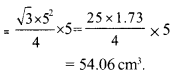Kerala Syllabus 9th Standard Maths Notes Chapter 11 Question 2.
A hexagonal hole of each side 2 metres is dug in the school ground to collect rainwater. It is 3 metres deep. It now has water one metre deep. How much litres of water is in it?
One side of the hexagon = 2 m
It is given that the depth of the pit is 3 metre but the water level is only in 1 metre. So we take the height as 1 metre.
Volume of water = Volume of the hexagonal prism = Area of the hexagon × heightArea of the regular hexagon is equal to six times the area of equilateral triangle.

Kerala Syllabus 9th Standard Maths Solutions Chapter 11 Question 3.
A hollow prism of base a square of side 16 centimetres contains water 10 centimetres high. If a solid cube of side 8 centimetres is immersed in it, by how much would the water level rise?
When a solid cube is immersed into water, the volume of water is raised.
Sum of the volume of the water at first time and volume of the solid cube immersed equally to the product of base area and height of water level now.
Volume of the water at first = Base area × height
= 16 × 16 × 10 = 2560 cm3
Volume of the solid cube when edges are 8 cm
= 8 × 8 × 8 = 512 cm3
Height at first = 10 cm
Water level raised = 12 -10 = 2 cm

Textbook Page No. 174

Class 9 Maths Solution Kerala Syllabus Chapter 11 Question 1.
The base of a prism is an equilateral triangle of perimeter 12 centimetres and its height is 5 centimetres. What is its total surface area?
Base perimeter of equilateral triangular prism = 12 cm
Base side = 12/3 = 4 cm
Lateral surface area of equilateral trian-gular prism = Base perimeter × Height
=12 × 5 = 60 cm2
Base area of equilateral triangular prism = $$\frac { √3 }{ 4 }$$ × 42 = 4√3 = 6.92 cm2
Total surface area of equilateral triangular prism =60 + 2 × 6.92 = 73.84 cm2

Kerala Syllabus 9th Standard Notes Maths Chapter 11 Question 2.
Two identical prisms with right tri-angles as base are joined to form a rectangular prism as shown below: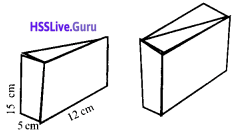What is total surface area?
The sides of the rectangular prism are
Length = 12 cm
Height = 15 cm.
Base area = 2 × perimeter of rectangle
2 × 12 × 5 = 120 cm2
Lateral surface area = base perimeter × height
= 2 (length + breadth) × height = 2(12 + 5) × 15
= 2 × 17 × 15 = 510 cm2
Total surface area = 120 + 510 = 630 cm2

Class 9 Maths Notes Kerala Syllabus Chapter 11 Question 3.
A water trough in the shape of a prism has trapezoidal faces. The dimensions of a base are shown in this picture:The length of the trough is 80 centimetres. It is to be painted inside and outside. How much would be the cost at 100 rupees per square metre?
Now we add the area of two edge faces and three lateral faces ( 1 on bottom and 2 on sides).
In figure, AB = 50 cm, CD = 70 cm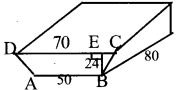EC = $$\frac { 70 – 50 }{ 2 }$$ = 10 cm
BE = 24 cm
BC2 = BE2 + EC2 = 242 + 102
= 576 + 100 = 676
BC = √676 = 26 cm.
Area of the trapezoidal faces of the water= 12 × 120 = 1440 cm2 Area of two trapezoidal faces
= 2 × 1440 = 2880 cm2 Area of two rectangular faces on sides
= 2 × 80 × 26=4160 cm2
Area of rectangular face at bottom
=50 × 80 = 4000 cm2
Total Area= 2880 + 4160 + 4000 = 11040 cm2 = 1.1 m2
Total area to be painted
= 2 × 1.1 =2.2 m2
Total cost = 2.2 × 100 = 220
= Rs. 220

Textbook Page No. 176

9th Standard Maths Notes Kerala Syllabus Chapter 11 Question 1.
The base radius of an iron cylinder is 15 centimetres and its height is 32 centimetres. It is melted and re-cast into a cylinder of base radius 20 centimetres. What is the height of this cylinder?
Volume of the first cylinder
= π R2 H = π (15)2 x 32 = 7200 π
Volume of the melted and recast cylinder = π r2h = π (20)2 x h = 400 π h
Volume remains constant when melted.
400 π h=7200π
h = $$\frac { 7200π }{ 400π }$$ =18
Height of the second cylinder = 18 cm

9th Class Maths Notes Malayalam Medium Chapter 11 Question 2.
The base radii of two cylinders of the same height are in the ratio 3:4. What is the ratio of their volumes?
Let r1 r2 be the radii of two cylinders,
then r1 : r2 = 3 : 4,Kerala Syllabus 9 Standard Maths Chapter 11 Question 3.
The base radii of two cylinders are in the ratio 2:3 and their heights are in the ratio 5:4.
i. What is the ratio of their volu-mes?
ii. The volume of the first cylinder is 720 cubic centimetres. What is the volume of the second?
i. If the base radius of the first cylinder is 2r then base radius of the second one is 3r.
Height of the first cylinder is 5h and second cylinder is 4h.
Volume of the first cylinder
= Base area × height .
= π × 2r × 2r × 5h = 20 π r2 h.
Volume of the second cylinder
= π × 3r × 3r × 4h=36 πr2h.
The ratio between the volumes
= 20πr2h : 36πr2h = 20 : 36.= 5 : 9

ii. The volume of the first cylinder is 720 cm3 is given. Let consider the volume of the second cylinder be x, then the ratio between the volumes is 5: 9
5 : 9 = 720 : x
5 × x = 9 × 720
x = 1296
Volume of the second cylinder = 1296 cm3

Textbook Page No. 178

Question 1.
The inner diameter of a well is 2.5 metres and it is 8 metres deep. What would be the cost of cementing its inside at 350 rupees per square metre?
Area of the part cemented = Base perimeter × height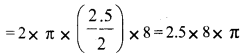= 20π = 20 × 3.14 = 62.8cm
Total cost of cementing = 62.8 × 350 = Rs. 21980

Question 2.
The diameter of a road roller is 80 centimetres and it is 1.20 metres long: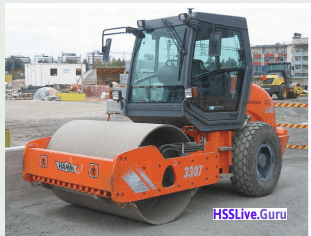What is the area of levelled surface, when it rolls once?
Radius of roller = 40 cm
Length of roller (height) = 1.20 m = 120 cm
Area of leveled surface, when it rolls once
= Curved surface area of the roller
= 2 × π × Radius × Height
= 2 × 3.14 × 40 × 120 = 30144 cm2 = 3.0144 m2

Question 3.
The base area and the curved surface area of a cylinder are equal. What is the ratio of the base radius and height?
Curved surface area of the cylinder = Base perimeter x height = 2 πrh
Base perimeter = πr2
If it is equal, 2 π rh = πr2
2rh = r × r, 2h = r
i.e., The radius is twice the height.

### Prisms Exam oriented Questions and Answers

Question 1.
A cylinder has base radius 4 cm and height 10 cm. Then find its lateral surface area.
Perimeter of a circle with radius 4 cm = 2 × π × 4 = 8π cm.
Curved surface area of the cylinder = Base perimeter × height = 8 π × 10= 80 π cm2

Question 2.
The volume and base area of a square prism are 3600 cubic centimetre and 144 cm2 respectively. What is its total surface area ?
Volume of the prism = 3600 cm3
Base area × height = 3600 cm3
144 × height = 3600
height = 3600/144 = 25 cm
Surface area = = Base area + Lateral surface area
Lateral surface area = Base perimeter × height
= 12 × 4 × 25 = 1200 cm2
(Base area = 144, One side = √144 =12 cm)
Total surface area = 144 + 1200 = 1344 cm2

Question 3.
A cylinder has height 20 cm and base radius 4 cm. Then find its vol-ume.
When the square of the radius is multiplied by π we get the area of the circle.
Base area of the cylinder
= π × 42 = 16 π cm2
The height of the cylinder is 20cm Volume = 16 π × 20 = 320 π cm3

Question 4.
The base edge of a square prism is 15 cm. The total surface area is 1950 m2.
i. What is its height ?
ii. Calculate the volume.
i .Base edge = 15 cm
Total surface area = Base area + Lateral surface area
= 2a2 + 4ah (base egde is ‘a’)
1950 = 2 × 152 + 4 × 15 × h
1950 = 450 + 60h
60h = 1950 – 450 = 1500
h = 1500/60 = 25 cm

ii. Volume = a2 × h = 15 × 15 × 25
= 5625 cm3

Question 5.
A square-shaped plot has length 24 m. A pond of 4 m length, 3 m breadth and 1.5 m height is dug here. If the sand dug out is levelled equally in the remaining area of the plot. Find the height of the levelled sand.
Area of the land
= 32 × 24
= 768 m2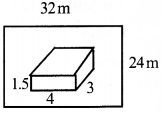Area of the pond = 4 × 3 = 12m2
Area of the remaining places = 768 – 12 = 756 m2.
Volume of the sand dug out = 4 × 3 × 1.5
Height of soil = $$\frac { Volume }{ Base area }$$
= $$\frac { 4 × 3 × 1.5 }{ 756 }$$ = 0.0238m = 2.38 cm

Question 6.
Two Aluminium sheets of length 10 cm and breadth 6 cm are folded to make two cylindrical vessels. One is made by folding lengthwise and the other breadthwise, which will have maximum volume?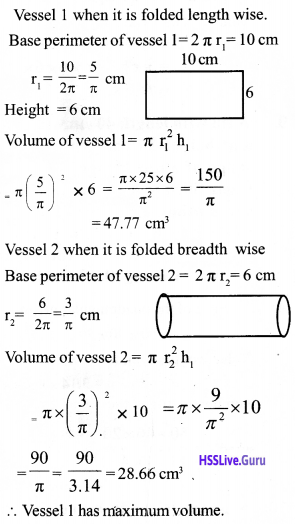Question 7.
A cylinder made of metal has radius 18 cm and height 40 cm. When this melts how many cylinders can be made of radius 2 cm and height 5 cm?
Volume of the first c ylinder = π r2H = π (18)2 × 40 = 12960 π cm3
Volume of the newly made cylinder = π (2)2 × 5 = 20π cm3
Number of the newfy made cylinders
= $$\frac { 12360π }{ 20π }$$ = 648 cylindres

Question 8.
If a wooden piec e is in the shape of square prism has base 12 cm and height 70 cm. What is the maximum volume of the cylinder that can be carved out of it?
Diameter of the largest cylinder =12cm Radius = 6 cm, Height = 70 cm
Volume of the cylinder
= base area × height
= πr2h = π × 6 × 6 × 70 = 7912.8 cm3

Question 9.
A tin with length 40 cm width 20 cm and height 20 cm, which is in the shape of a quadrangular prism has sugar-filled in it. If the sugar is measured using a cylindrical vessel with radius 4 cm and height 15 cm, then how many times can the sugar be measured using the vessel?
Volume of the quadrangular prism = 50 × 40 × 20
Volume of the cylinder = πr2h.
= 3.14 × 4 × 4 × 15
Number of times the sugar can be measured = $$\frac { 50 × 40 × 20 }{ 3.14 × 4 × 4 × 15 }$$ = 53 times

Question 10.
A prism is made by cutting cardboard as shown in the figure.a. What is the name of the prism?
b. What will be the area of the required cardboard for making the rectangle form?
a.Triangular prism
b. Lateral area = base perimeter × height = (15 + 13 + 14) × 20 = 42 × 20
= 840 cm2

Question 11.
Two tins in the shape of cylinder has radii 15 cm and 10 cm respectively. The heights are 25 cm and 18 cm respectively.In the both cylinders, the ghee are filled. When it transferred into another cylindrical-shaped tin. If there is ghee in the bigger tin with height 30 cm. Then find its radius.
‘Volume of the ghee in the first tin
= πr2h = π × 15 × 15 × 25 = 5625π cm3
Volume of the ghee in the second tin
= πr2h = π × 10 × 10 × 18 = 1800 π cm3
Total volume = 5625 π + 1800 π
=7425 π cm3 Volume of the ghee in the bigger tin
= πr2h = 7425 π
h =30
πr2h = πr2 × 30 = 7425 π
r2 = $$\frac { 7425 π }{ 30π }$$ = 247.5
$$r=\sqrt{247.5}=15.73 \mathrm{cm}$$

Question 12.
The diameter of a water tank in the shape of a cylinder is 3 m and height 4 m. How many litres of water will the tank hold?
Radius = 1.5 m, Height = 4m
Volume of the cylinder
= Base area × height = πr2h = 3.14 × 1.5 × 1.5 × 4 = 28.26 m3
= 28.26 × 100 × 100 × 100 cm3
= 28260000 cm3 = 28260000/1000 = 28260 liter

Question 13.
If a box in the shape of a square prism has length 25 cm, 20 cm width and 7-litre volume. What will be its height?
Volume = 7 litre = 7000 cm3
lbh = 7000
25 × 20 × h = 7000
h = $$\frac { 7000 }{ 25 × 20 }$$ = 14 cm

Question 14.
If the base length, width, height of a quadrangular prism are 37.5 cm, 18 cm, 40 cm respectively. Find the area of cube which has same volume as that of this prism.
Volume of quadrangular prism = base area × height = 37.5 × 18× 40 = 27000 cm3
Volume of quadrangular prism = Volume of cube
∴ Volume of cube = 27000 cm3
Let one side of a cube be x, then a3 = 27000 ; a = 30cm
30 × 30 × 30 = 27000, Hence
Surface area of the cube = 6a2 = 6 × 30 × 30 = 5400 cm2

Question 15.
The diameters of two-cylinder are in the ratio 2 : 3 and their heights in the ratio 5: 4. If the volume of the first cylinder is 400 cm3, then find the volume of the second cylinder.
x = $$\frac { 400 × 9 }{ 5 }$$ = 80 × 9 = 720 cm3